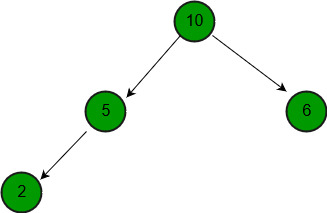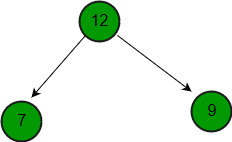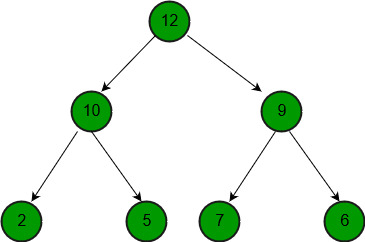# Merge two binary Max Heaps

• Difficulty Level : Easy
• Last Updated : 04 Oct, 2021

Given two binary max heaps as arrays, merge the given heaps.
Examples :

```Input  : a = {10, 5, 6, 2},
b = {12, 7, 9}
Output : {12, 10, 9, 2, 5, 7, 6}```Attention reader! Don’t stop learning now. Get hold of all the important DSA concepts with the DSA Self Paced Course at a student-friendly price and become industry ready.  To complete your preparation from learning a language to DS Algo and many more,  please refer Complete Interview Preparation Course.

In case you wish to attend live classes with experts, please refer DSA Live Classes for Working Professionals and Competitive Programming Live for Students.

` `` `` `

The idea is simple. We create an array to store result. We copy both given arrays one by one to result. Once we have copied all elements, we call standard build heap to construct full merged max heap.

## C++

 `// C++ program to merge two max heaps.``#include ``using` `namespace` `std;` `// Standard heapify function to heapify a``// subtree rooted under idx. It assumes``// that subtrees of node are already heapified.``void` `maxHeapify(``int` `arr[], ``int` `n, ``int` `idx)``{``    ``// Find largest of node and its children``    ``if` `(idx >= n)``        ``return``;``    ``int` `l = 2 * idx + 1;``    ``int` `r = 2 * idx + 2;``    ``int` `max;``    ``if` `(l < n && arr[l] > arr[idx])``        ``max = l;``    ``else``        ``max = idx;``    ``if` `(r < n && arr[r] > arr[max])``        ``max = r;` `    ``// Put maximum value at root and``    ``// recur for the child with the``    ``// maximum value``    ``if` `(max != idx) {``        ``swap(arr[max], arr[idx]);``        ``maxHeapify(arr, n, max);``    ``}``}` `// Builds a max heap of given arr[0..n-1]``void` `buildMaxHeap(``int` `arr[], ``int` `n)``{``    ``// building the heap from first non-leaf``    ``// node by calling max heapify function``    ``for` `(``int` `i = n / 2 - 1; i >= 0; i--)``        ``maxHeapify(arr, n, i);``}` `// Merges max heaps a[] and b[] into merged[]``void` `mergeHeaps(``int` `merged[], ``int` `a[], ``int` `b[],``                ``int` `n, ``int` `m)``{``    ``// Copy elements of a[] and b[] one by one``    ``// to merged[]``    ``for` `(``int` `i = 0; i < n; i++)``        ``merged[i] = a[i];``    ``for` `(``int` `i = 0; i < m; i++)``        ``merged[n + i] = b[i];` `    ``// build heap for the modified array of``    ``// size n+m``    ``buildMaxHeap(merged, n + m);``}` `// Driver code``int` `main()``{``    ``int` `a[] = { 10, 5, 6, 2 };``    ``int` `b[] = { 12, 7, 9 };` `    ``int` `n = ``sizeof``(a) / ``sizeof``(a);``    ``int` `m = ``sizeof``(b) / ``sizeof``(b);` `    ``int` `merged[m + n];``    ``mergeHeaps(merged, a, b, n, m);` `    ``for` `(``int` `i = 0; i < n + m; i++)``        ``cout << merged[i] << ``" "``;` `    ``return` `0;``}`

## Java

 `// Java program to merge two max heaps.` `class` `GfG {` `    ``// Standard heapify function to heapify a``    ``// subtree rooted under idx. It assumes``    ``// that subtrees of node are already heapified.``    ``public` `static` `void` `maxHeapify(``int``[] arr, ``int` `n,``                                             ``int` `i)``    ``{``        ``// Find largest of node and its children``        ``if` `(i >= n) {``            ``return``;``        ``}``        ``int` `l = i * ``2` `+ ``1``;``        ``int` `r = i * ``2` `+ ``2``;``        ``int` `max;``        ``if` `(l < n && arr[l] > arr[i]) {``            ``max = l;``        ``}``        ``else``            ``max = i;``        ``if` `(r < n && arr[r] > arr[max]) {``            ``max = r;``        ``}``        ` `        ``// Put maximum value at root and``        ``// recur for the child with the``        ``// maximum value``        ``if` `(max != i) {``            ``int` `temp = arr[max];``            ``arr[max] = arr[i];``            ``arr[i] = temp;``            ``maxHeapify(arr, n, max);``        ``}``    ``}``    ` `    ``// Merges max heaps a[] and b[] into merged[]``    ``public` `static` `void` `mergeHeaps(``int``[] arr, ``int``[] a,``                                  ``int``[] b, ``int` `n, ``int` `m)``    ``{``        ``for` `(``int` `i = ``0``; i < n; i++) {``            ``arr[i] = a[i];``        ``}``        ``for` `(``int` `i = ``0``; i < m; i++) {``            ``arr[n + i] = b[i];``        ``}``        ``n = n + m;` `        ``// Builds a max heap of given arr[0..n-1]``        ``for` `(``int` `i = n / ``2` `- ``1``; i >= ``0``; i--) {``            ``maxHeapify(arr, n, i);``        ``}``    ``}``    ` `    ``// Driver Code``    ``public` `static` `void` `main(String[] args)``    ``{``        ``int``[] a = {``10``, ``5``, ``6``, ``2``};``        ``int``[] b = {``12``, ``7``, ``9``};``        ``int` `n = a.length;``        ``int` `m = b.length;` `        ``int``[] merged = ``new` `int``[m + n];` `        ``mergeHeaps(merged, a, b, n, m);` `        ``for` `(``int` `i = ``0``; i < m + n; i++)``            ``System.out.print(merged[i] + ``" "``);``        ``System.out.println();``    ``}``}`

## Python3

 `# Python3 program to merge two Max heaps.` `# Standard heapify function to heapify a``# subtree rooted under idx. It assumes that``# subtrees of node are already heapified.``def` `MaxHeapify(arr, n, idx):``    ` `    ``# Find largest of node and``    ``# its children``    ``if` `idx >``=` `n:``        ``return``    ``l ``=` `2` `*` `idx ``+` `1``    ``r ``=` `2` `*` `idx ``+` `2``    ``Max` `=` `0``    ``if` `l < n ``and` `arr[l] > arr[idx]:``        ``Max` `=` `l``    ``else``:``        ``Max` `=` `idx``    ``if` `r < n ``and` `arr[r] > arr[``Max``]:``        ``Max` `=` `r` `    ``# Put Maximum value at root and``    ``# recur for the child with the``    ``# Maximum value``    ``if` `Max` `!``=` `idx:``        ``arr[``Max``], arr[idx] ``=` `arr[idx], arr[``Max``]``        ``MaxHeapify(arr, n, ``Max``)` `# Builds a Max heap of given arr[0..n-1]``def` `buildMaxHeap(arr, n):``    ` `    ``# building the heap from first non-leaf``    ``# node by calling Max heapify function``    ``for` `i ``in` `range``(``int``(n ``/` `2``) ``-` `1``, ``-``1``, ``-``1``):``        ``MaxHeapify(arr, n, i)` `# Merges Max heaps a[] and b[] into merged[]``def` `mergeHeaps(merged, a, b, n, m):``    ` `    ``# Copy elements of a[] and b[] one``    ``# by one to merged[]``    ``for` `i ``in` `range``(n):``        ``merged[i] ``=` `a[i]``    ``for` `i ``in` `range``(m):``        ``merged[n ``+` `i] ``=` `b[i]` `    ``# build heap for the modified``    ``# array of size n+m``    ``buildMaxHeap(merged, n ``+` `m)` `# Driver code``if` `__name__ ``=``=` `'__main__'``:``    ``a ``=` `[``10``, ``5``, ``6``, ``2``]``    ``b ``=` `[``12``, ``7``, ``9``]` `    ``n ``=` `len``(a)``    ``m ``=` `len``(b)` `    ``merged ``=` `[``0``] ``*` `(m ``+` `n)``    ``mergeHeaps(merged, a, b, n, m)` `    ``for` `i ``in` `range``(n ``+` `m):``        ``print``(merged[i], end ``=` `" "``)` `# This code is contributed by PranchalK`

## C#

 `// C# program to merge two max heaps.``using` `System;` `class` `GfG {` `    ``// Standard heapify function to heapify a``    ``// subtree rooted under idx. It assumes``    ``// that subtrees of node are already heapified.``    ``public` `static` `void` `maxHeapify(``int``[] arr,``                                  ``int` `n, ``int` `i)``    ``{``        ``// Find largest of node``        ``// and its children``        ``if` `(i >= n) {``            ``return``;``        ``}``        ``int` `l = i * 2 + 1;``        ``int` `r = i * 2 + 2;``        ``int` `max;``        ``if` `(l < n && arr[l] > arr[i]) {``            ``max = l;``        ``}``        ``else``            ``max = i;``        ``if` `(r < n && arr[r] > arr[max]) {``            ``max = r;``        ``}``        ` `        ``// Put maximum value at root and``        ``// recur for the child with the``        ``// maximum value``        ``if` `(max != i) {``            ``int` `temp = arr[max];``            ``arr[max] = arr[i];``            ``arr[i] = temp;``            ``maxHeapify(arr, n, max);``        ``}``    ``}` `    ``// Merges max heaps a[] and b[] into merged[]``    ``public` `static` `void` `mergeHeaps(``int``[] arr, ``int``[] a,``                                  ``int``[] b, ``int` `n, ``int` `m)``    ``{``        ``for` `(``int` `i = 0; i < n; i++) {``            ``arr[i] = a[i];``        ``}``        ``for` `(``int` `i = 0; i < m; i++) {``            ``arr[n + i] = b[i];``        ``}``        ``n = n + m;` `        ``// Builds a max heap of given arr[0..n-1]``        ``for` `(``int` `i = n / 2 - 1; i >= 0; i--) {``            ``maxHeapify(arr, n, i);``        ``}``    ``}``    ` `    ``// Driver Code``    ``public` `static` `void` `Main()``    ``{``        ``int``[] a = {10, 5, 6, 2};``        ``int``[] b = {12, 7, 9};``        ``int` `n = a.Length;``        ``int` `m = b.Length;` `        ``int``[] merged = ``new` `int``[m + n];` `        ``mergeHeaps(merged, a, b, n, m);` `        ``for` `(``int` `i = 0; i < m + n; i++)``            ``Console.Write(merged[i] + ``" "``);``        ``Console.WriteLine();``    ``}``}` `// This code is contributed by nitin mittal`

## Javascript

 ``

Output:

`12 10 9 2 5 7 6`

Since time complexity for building the heap from array of n elements is O(n). The complexity of merging the heaps is equal to O(n + m).
This article is contributed by K Akhil Reddy. If you like GeeksforGeeks and would like to contribute, you can also write an article using write.geeksforgeeks.org or mail your article to review-team@geeksforgeeks.org. See your article appearing on the GeeksforGeeks main page and help other Geeks.